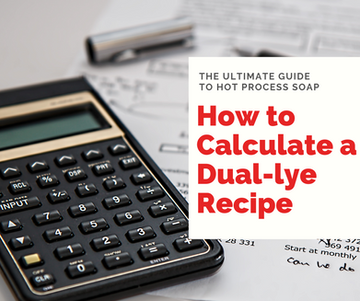top of pageSearch

# How to Calculate a Dual-Lye Recipe"How do I calculate a dual-lye recipe?" The most common and obvious answer is "Use a calculator." Well duh, why didn't I think of that? One of the most popular soap calculator websites, and one of the select few that offers a dual-lye option, is no longer functioning and has left soap makers across the globe scrambling to try and figure out what to do.

Of course there are other soap calculators available, but Soapee was a personal favorite and the soap calculator that we used to teach our students with, not to mention most of the other calculators don't have a dual-lye option. So, what can we do? Is there a way can we calculate this ourselves? Let's find out!

To quickly calculate the amount of lye necessary for a dual-lye recipe without the dual-lye function in a soap calculator, we need to calculate two things: the amount of NaOH needed to saponify the recipe and the amount of KOH needed to saponify the recipe. We can then use this information to determine our required lye amounts. Let's use a simple 100% coconut oil recipe as an example:

Our sample recipe requires 10 ounces (283.5g) of coconut oil.

1. Using any soap calculator, select the option to make bar soap, using NaOH as the lye. In order to completely saponify our sample recipe with NaOH, we need to use 1.83 ounces of NaOH (51.93 g). Write this number down.

2. We would then calculate the recipe as a liquid soap made with KOH. To saponify our 10 ounces of coconut oil, we need to use 2.86 ounces (80.95g) of KOH. Write this number down.

3. For our final step, we need to calculate the percentages or ratio of lyes we would like to use. Let's pretend we want to make a liquid soap with 80% KOH and 20% NaOH. How would we determine that?

Well, we know that to saponify 100% of our recipe with NaOH, we would use 1.83 ounces (51.93 g) of NaOH. To saponify 20% of the recipe with NaOH, we would take our total recipe oils and multiply by 20% or 0.2.

We also know that to saponify 100% of our recipe with KOH, we would use 2.86 ounces (80.95g) of KOH. To saponify 80% of the recipe with KOH, we would take our total recipe oils and multiply by 80% or 0.8.

Let's do the math:

20% NaOH= 1.83 ounces (51.93 g) x 0.2= 0.37 ounces (10.39g)

80% KOH= 2.86 ounces (80.95g) x 0.8= 2.28 ounces (64.76g)

That's it! Easy peasy. Soap calculators compute dual-lye recipes the same way. Now if you are ever in a pinch and need to quickly and easily calculate a dual-lye recipe, you can! Try it out- Use the recipe above to create a dual-lye soap made with 60% NaOH and 40% KOH. How much of each would you use?

For those of you die-hard Soapee fans like myself, while it is down, I recommend Soap Making Friend. They have a dual-lye soap calculator and offer an easy-to-use format. Their website can be found at www.soapmakingfriend.com

bottom of page